Students get through the MP Board Class 11th Physics Important Questions Chapter 4 Motion in a Plane which are most likely to be asked in the exam.

## MP Board Class 11th Physics Important Questions Chapter 4 Motion in a Plane

Motion in a Plane Class 11 Important Questions Very Short Answer Type

Question 1.
State for each of the following physical quantities, if it is a scalar or a vector : Volume, mass, speed, acceleration, density, number of moles, velocity, angular
frequency, displacement, angular velocity.
Scalar quantities : Volume, mass, speed, density, number of moles, angular frequency.
Vector quantities : Acceleration, velocity, displacement, angular velocity.

Question 2.
Pick out the only vector quantity in the following list:
Temperature, pressure, impulse, time, power, total path, length, energy, gravitational potential, coefficient of friction, charge.
Impulse. As impulse = force × time = change in momentum . As force and momentum are vector quantities, so it is a vector.

Question 3.
What do you mean by magnitude of a vector quantity?
The positive value of the modulus of a vector quantity is called magnitude of
vector quantity. It is represented by $$|\vec{a}|$$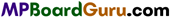Question 4.
Whether addition of two vectors depends upon the order of placement of the vectors.
No, according to the commutative law of vector addition $$\vec{a}+\vec{b}$$ = $$\vec{b}+\vec{a}$$.

Question 5.
Can two vectors of different dimension can be added?
No, we can add two vectors of same dimension.

Question 6.
How can a vector and scalar be added?
A vector and a scalar cannot be added.

Question 7.
How can three vectors be added so that its resultant is zero?
If three vectors can be represented completely by the three sides of a triangle taken in the same order, then their resultant is zero.

Question 8.
What do you mean by unit vector and zero vector?
If the magnitude of a vector is one then it is called as unit vector and if its
magnitude is zero and direction is uncertain is called zero vector, unit vector
$$\hat{a}=\frac{\vec{a}}{|\vec{a}|}$$

Question 9.
What do you mean by resolution of a vector?
When a vector is divided into two or more components such that their addition is the same vector then it is called resolution of vector.Question 10.
Can the magnitude of component of vector more than its original vector?
No, it cannot be.

Question 11.
Can a vector be multiplied with a scalar? Illustrate with example.
Yes, $$\vec{F}=m \cdot \vec{a}$$

Question 12.
When the scalar product of two vectors is maximum?
When both the vectors are parallel, then angle between them is 0° or 180°. Then scalar product will be maximum.

Question 13.
Area of a surface is vector or scalar quantity?
It is vector quantity because its direction is perpendicular to its surface.

Question 14.
Which type of quantity is modulus of a vector?
Modulus of a vector is scalar quantity.

Question 15.
What do you mean by displacement vector?
Displacement vector is those vector which specify that how much and in which direction an object has displaced in time intervel t1 and t2.

Question 16.
What do you mean by equal vectors?
Two vectors whose magnitude and directions are same are called equal vectors.

Question 17.
Write the triangle law of vector addition.
Triangle law of vector addition : If two vectors are represented by the adjacent sides of a triangle in a definite order. Then the
resultant will be given by the third side taken in the opposite manner.
i.e., $$\vec{R}=\overrightarrow{O A}+\overrightarrow{A B}$$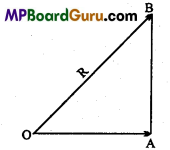Question 18.
Can a vector of IS dyne be added in a vector of 10 Newton?
Yes, it can be added because dimensions of both physical quantities are same.Question 19.
Why displacement vector and velocity vector cannot be added?
They cannot be added because their dimensions are different.

Question 20.
What will happen if a vector is extended in its parallel direction?
No changes will occur in vector.

Question 21.
When the addition of two vectors are maximum and minimum?
When both the vectors are parallel the addition is maximum and when they are opposite their addition is minimum.

Question 22.
Can the resultant of two different vectors be zero?
No, because the minimum magnitude of $$\vec{A}+\vec{B}$$ is A – B.

Question 23.
Whether addition of vectors depends on order they placed?
No.

Question 24.
If P and Q are two vector quantities then PQ will be which quantity?
It is a scalar quantity.

Question 25.
When vector product of two vectors is maximum?
When two vectors are perpendicular to each other, then their vector product is maximum.

Question 26.
If a component of a vector is zero and other component of same vector is non-zero, can the magnitude of that vector be zero?
No.

Question 27.
If the angle between two vectors are changed keeping magnitude to be same; then what will be the effect on resultant vector?
Magnitude and direction of resultant vector will get changed.Question 28.
When addition and subtraction of two vectors are equal?
When the magnitude of both vectors are same and mutually perpendicular to each other then addition and subtraction of two vectors will be equal.

Question 29.
If $$\vec{A} \cdot \vec{C}=\vec{B} \cdot \vec{C}$$ whether $$\vec{A}$$ and $$\overrightarrow{\boldsymbol{B}}$$ will be always equal?
No.

Question 30.
What is projectile motion? When does the trajectory is parabola?
When a body is projected at an angle other than vertical, the body moves under gravity and falls at different points on the earth. The path traced out by the body is called trajectory and its motion is called projectile.
Example : Motion of cannon ball, the object released from an aeroplane flying horizontally. Trajectory is parabola when air of friction is negligible.

Question 31.
At what angle the horizontal range is maximum?
When θ = 45°, = R max = $$\frac{u^{2}}{g}$$

Question 32.
From top of a tower two bullets are shot with different velocities in horizontal direction. Which one will reach on the ground first?
Both bullets will reach the ground at the same time because initial velocity in vertical direction is same for them.

Motion in a Plane Class 11 Important Questions Short Answer Type

Question 1.
Establish the following vector inequalities geometrically or otherwise :
(a) $$|a+b| \leq|a|+|b|$$
(b) $$|\boldsymbol{a}+\boldsymbol{b}| \geq\|\boldsymbol{a}|-| \boldsymbol{b}\|$$.
When does the equality sign above apply?
Let the two vectors $$\vec{a}$$ and $$\vec{b}$$ be represented by the sides OP and PQ of the ΔOPQ taken in the same order, then their resultant is represented by the side OQ of the triangle such that
OQ = a + b as shown in fig.
Thus, OP = a,PQ = b
∴ $$|O P|=|a|,|P Q|=|b|$$
and $$|O Q|=|a+b|$$
(a) $$|a+b| \leq|a|+|b|$$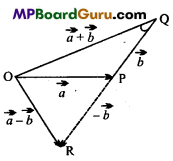Proof:
We know from the property of a triangle that its one side is always less than the sum of the lengths of its two other sides.
Now in Δ OPQ,
OQ<OP+PQ
or $$|a+b|<|a|+|b|$$ …(1)
If the two vectors a and b are acting along the same straight line i.e., are collinear and in the same direction, then
θ (angle between them) = 0
∴ $$|a+b|=\sqrt{a^{2}+b^{2}+2 a b \cos \theta}=\sqrt{(a+b)^{2}}$$
= a + b = $$|a|+|b|$$ ..(2)
From eqns. (1) and (2), we get
$$|a+b| \leq|a|+|b|$$
The equality sign applies if a and b are collinear and act in same direction.
(b) $$\text { (b) }|a+b| \geq \| a|-| b||$$

Proof:
One side is more than the difference of two other sides i.e., in ΔOPQ in fig.
OQ >$$|O P-P Q|$$
The modulus of (OP – PQ) has been taken because L.H.S. is + ve and R.H.S. may be – ve if OP < PQ.
or $$|a+b|>\| a|-| b||$$ ….(3)
If a and b are acting along the same straight line in the opposite direction, then
$$|a+b|>\| a|-| b||$$
From eqns. (3) and (4), we get
$$|a+b| \geq \| a|-| b||$$
Equality sign holds for as stated in eqn. (4).Question 2.
Explain scalar product of two vectors?
Let the angle between two vectors $$\vec{A}$$ and $$\vec{B}$$ is θ
∴ Scalar product of $$\vec{A}$$ and $$\vec{B}$$ will be
$$\vec{A} \cdot \vec{B}$$ = AB cosθ

Example:
Let a force$$\vec{F}$$ is acting on a particle and is displaced through $$\vec{r}$$ at an angle θ then
$$\vec{F} \cdot \vec{r}$$ = Frcosθ
Now, $$\vec{A} \cdot \vec{B}$$ = AB cosθ
Hence, $$\vec{A} \cdot \vec{B}$$ = 0 if either $$|\vec{A}|$$ = 0 or $$|\vec{B}|$$= 0 or θ= 90°.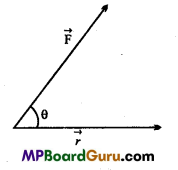Question 3.
What type of quantity is work?
Since work = Force × Displacement
i.e., W = $$\vec{F} \cdot \vec{d}$$
Since scalar product of two vectors are scalar, therefore work is a scalar quantity.

Question 4.
What type of quantity is kinetic energy?
K.E =$$\frac{1}{2}$$mv2 = $$\frac{1}{2}$$m $$(\vec{v} \cdot \vec{v})$$
Since, $$\vec{v} \cdot \vec{v}$$ will be a scalar quantity, so kinetic energy will be a scalar quantity.

Question 5.
Prove that addition of vectors obeys commutative law.
Or
State and prove the commutative law of vector addition.
Consider two vectors $$\vec{a}$$ and $$\vec{b}$$ which are in the same plane $$\overrightarrow{O A}=\vec{a}$$ and $$\overrightarrow{A B}=\vec{b}$$.
Join $$\overrightarrow{O B}$$.
$$\vec{a}+\vec{b}=\overrightarrow{O A}+\overrightarrow{A B}=\overrightarrow{O B}$$ …(1)
Complete the parallelogram OABC. In a parallelogram, op¬posite sides are parallel and equal. Hence,
$$\overrightarrow{A B}=\overrightarrow{O C}=\vec{b} \text { and } \overrightarrow{O A}=\overrightarrow{C B}=\vec{a}$$
$$\vec{b}+\vec{a}=\overrightarrow{O C}+\overrightarrow{C B}=\overrightarrow{O B}$$ …..(2)
From eqns. (1) and (2), $$\vec{a}+\vec{b}=\vec{b}+\vec{a}$$
Hence, the addition of vectors obey commutative law.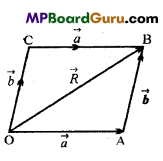Question 6.
Show that addition of vectors obey the associative law.
In a plane choose origin O and construct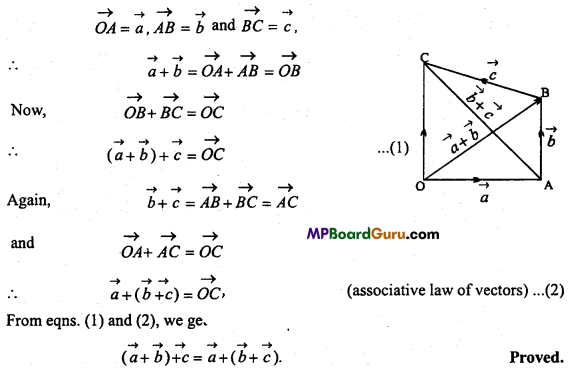Question 7.
Explain the law of parallelogram of vector’s addition.
If the two vectors acting at a point are represented in magnitude and direction by the adjacent sides of a parallelogram, then their resultant vector is represented in magnitude and direction by the diagonal passing through their common origin.
If two vectors $$\vec{A}$$ and $$\vec{B}$$ are represented by two adjacent sides of a parallelogram OACB, the diagonal OC represents the resultant of these two vectors.
In parallelogram OACB,
OB= AC
∴ $$\overrightarrow{O B}=\overrightarrow{A C}=\vec{B}$$
In ? OAC, as per triangle law of vector addition,
$$\overrightarrow{O C}=\overrightarrow{O A}+\overrightarrow{A C}$$
∴ $$\vec{R}=\vec{A}+\vec{B}$$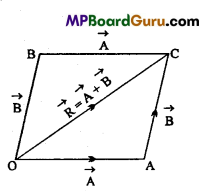Question 8.
Write the expression of position vector, velocity vector and acceleration vector for a particle moving in a plane.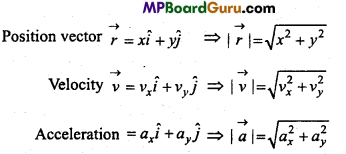Question 9.
What is the effect of air on the time of flight and range of projectile?
Time of flight and range will be decreased.

Question 10.
A food packet is released from an aeroplane flying horizontally. What will be the path of packet:
(i) Seen from the plane and
(ii) Seen from the earth?
(i) In a straight line vertically downward.
(ii) Parabolic.

Question 11.
An aeroplane is moving horizontally with uniform velocity. A bomb is dropped from the plane. What will be the position of plane, when the bomb strikes the ground?
When the friction of medium is zero, the plane will be just above the point where the bomb strike the ground.
When the friction is not zero, the plane will be little ahead above the point where the bomb will strike the ground.

Question 12.
What will be the change in centripetal force when speed of an object and radius of path are doubled in circular motion.
Centripetal force F = $$\frac{m v^{2}}{r}$$
When v1 = 2v and r1 = 2r
Then, F1 = $$\frac{m v_{1}^{2}}{r_{1}}$$
or F1 = $$\frac{m(2 v)^{2}}{2 r}=\frac{4 m v^{2}}{2 r}$$
or F1 = $$\frac{2 m v^{2}}{r}$$
or F1= 2F is centripetal force will get doubled.

Question 13.
What do you mean by uniform circular motion? Give two examples.
When a body moves in a circular path with constant speed, then its motion is called uniform circular motion.
Example: Motion of the tip of the blade of a fan, motion of the earth around the sun is nearly a uniform circular motion.Question 14.
What do you mean by angular displacement?
The angle suspended by the particle on the circular path in given time interval is called an angular displacement of the particle its unit is radian.

Question 15.
If the velocity of projectile motion is doubled then what will be the effect on its horizontal range?
since R = $$\frac{u^{2} \sin ^{2} \theta}{g}$$
i.e., R ∝ u2
When velocity of projectile motion is doubled then its horizontal range get four times increased.

Question 16.
What do you understand by time-period and frequency?
Time-period is defined as the time taken by an object to complete one rotation. Its unit is second. If the angular velocity of an object is a> and angle suspended at the centre during one complete rotation is 2π, then
Time-period T = $$\frac{2 \pi}{\omega}$$
or ω = $$\frac{2 \pi}{T}$$
Frequency is defined as the number of rotations completed by the body in one second. Its unit is hertz.
If time-period is T, then frequency n = $$\frac{1}{T}$$
or n = $$\frac{1}{2 \pi / \omega}=\frac{\omega}{2 \pi}$$
or ω = 2πn

Question 17.
Define linear velocity and angular velocity of a particle in circular motion. Establish the relation between them.
Angular velocity :
The rate of change of angle subtended by a body moving in a circular path, at the centre of the path per second is called angular velocity of the body.
Linear velocity :
The distance travelled by the particle on the circular path in one second is called linear velocity.
Its SI unit is ms-1.

Relation between linear velocity and angular velocity:
Let a particle is moving in a circular path of radius r and centre O. Its linear velocity is v and angular velocity is ω
If in small time Δt, the particle travels distance Δs on the circle,
∴ ∠POQ = Δθ
Hence’ ω = $$\frac{\Delta \theta}{\Delta t}$$ ….(1)
and ν = $$\frac{\Delta s}{\Delta t}$$ ….(2)
But, Angle = $$\frac{\text { Arc }}{\text { Radius }}$$
∴ Δθ = $$\frac{\Delta s}{r}$$
∴ From eqn. (1), we get
ω = $$\frac{\Delta s}{r \Delta t}$$
or $$\frac{\Delta s}{\Delta t}$$ = ωr
∴ ν = ωr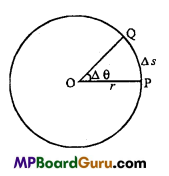Hence, Linear velocity = Angular velocity × Radius.

Question 18.
Define centripetal acceleration and derive its expression. Also deduce its direction.
Centripetal acceleration :
It is defined as the acceleration which always acts towards the centre along the radius of the circular path.
“Centripetal” comes from a Greek term which means “centre seeking”.
Expression for centripetal acceleration:
Let a body be moving with constant angular velocity? and constant speed? in a circular path of radius R and centre O.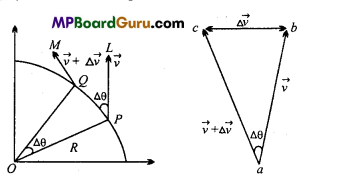Let the body be at points P and Q at time t and t + Δt respectively.
Also let Δθ= ∠POQ be the angular displacement in a small time interval Δt, thus
ω = $$\frac{\Delta \theta}{\Delta t}$$ = or Δθ = ωΔt …(1)
If ν and $$\vec{v}+\Delta \vec{v}$$be the velocity vectors of the body at points P and Q acting along PL and QM respectively. As the body is moving with uniform speed, so lengths PL and PM are equal i.e., PL = PM. The change in velocity $$\Delta \vec{v}$$ from P to Q is due to the change in the direction of velocity vector.
Hence magnitude of acceleration of the body is given by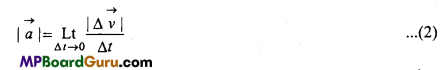From point’a’draw $$\overrightarrow{a b}$$ parallel and equal to $$\overrightarrow{P L}$$ and $$\overrightarrow{a c}$$ parallel and equal to $$\overrightarrow{Q M}$$,
thus
$$\overrightarrow{a b}=\vec{v}, \overrightarrow{a c}=\vec{v}+\Delta \vec{v}$$
∴ $$\overrightarrow{b c}=\Delta \vec{v}$$
Clearly the angle between $$\overrightarrow{a b}$$ and $$\overrightarrow{a c}$$ is Δθ . As Δt is quite small so a and c lie very close to each other, thus be can be taken to be an arc of $$\widehat{b c}$$ a circle of radius
ab = $$|\vec{v}|$$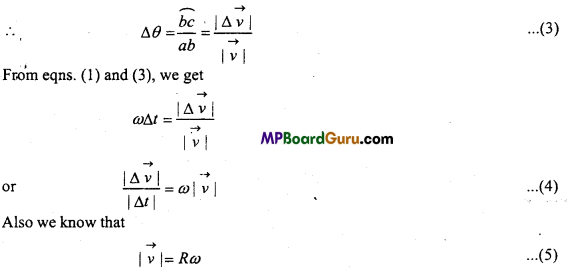Also when Δt → 0, then L.H.S. of eqn. (4) represents acceleration. Thus eqn. (4) can be written as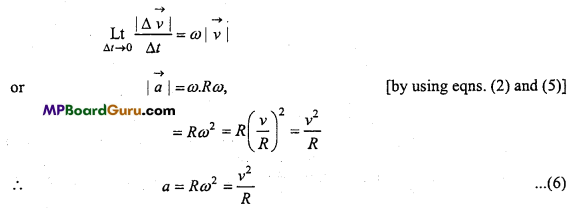Eqn. (6) gives the magnitude of the acceleration produced in the body.
Direction of acceleration :
Acceleration always acts in the direction of Δν which acts from b to c. When Δt is decreased, Δθ also decreases. If Δt → 0, then Δθ → 0, so Δν tends to be perpendicular in ab. As ab || PL, so Δν tends to act perpendicular to PL i.e., along PO is along the radius towards the centre of circular path.
Since ν and R are always constant, so magnitude of centripetal acceleration is also constant. But the direction changes pointing always towards the centre. So centripetal acceleration is not a constant vector.Motion in a Plane Class 11 Important Questions Long Answer Type

Question 1.
State parallelogram law of vector addition of two vectors and derive an expression for their resultant vector.
Ans. Parallelogram law of vector addition : If the two vectors acting at a point are represented in magnitude and direction by the adjacent sides of a parallelogram, then their resultant vector is represented in magnitude and direction by the diagonal passing through their common origin.
If two vectors $$\vec{A}$$ and $$\vec{B}$$ are represented by two adjacent sides of a parallelogram OACB, the diagonal OC represents the resultant of these two vectors.
In parallelogram OACB,
OB= AC
∴ $$\overrightarrow{O B}=\overrightarrow{A C}=\vec{B}$$
In Δ OAC, as per triangle law of vector addition,
$$\overrightarrow{O C}=\overrightarrow{O A}+\overrightarrow{A C}$$
∴ $$\vec{R}=\vec{A}+\vec{B}$$Two vectors $$\vec{a}$$ and $$\vec{b}$$ are completely represented by the two sides OA and OB of a parallelogram respectively as shown in fig.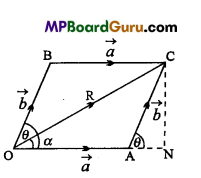The angle between two vectors $$\vec{a}$$ and $$\vec{b}$$ is θ. The diagonal
OC of the paralellogram gives the resultant $$\vec{R}$$,
Such that $$\vec{R}$$ = $$\vec{R}=\vec{a}+\vec{b}$$
The resultant $$\vec{R}$$ makes a angle with direction of $$\vec{a}$$ From point C draw a perpendicular CN on extended OA.
In ΔANC,
sinθ = $$\frac{C N}{A C}$$ ⇒ CN= AC sinθ
⇒ CN = b sinθ ….(1)
Also, cosθ = $$\frac{A N}{A C}$$
⇒ AN = AC cosθ = b cosθ ….(2)
∴ ON = OA + AN =a+b cosθ ….(3)
In ΔONC, OC2 = ON2 + CN2
⇒ R2 = (a +b cosθ)2 +(bsin?)2
or R2 = a2 +b2 cos2 θ+2abcosθ+b2 sin2θ
or R2 = a2 +b2(sin2θ+ cos2 θ) + 2abcosθ
or R2 = a2 +b2 +2abcosθ
or R = $$\sqrt{a^{2}+b^{2}+2 a b \cos \theta}$$ …(4)
The above eqn. (4) is the required expression.
Direction of R is given by
tanα = $$\frac{b \sin \theta}{a+b \cos \theta}$$ or a = tan-1$$\left(\frac{b \sin \theta}{a+b \cos \theta}\right)$$

Question 2.
What do you mean by two-dimensional motion? Establish the relation between displacement, velocity and acceleration.
TWo-dimensional motion: When a body moves in a plane, its motion is called two-dimensional motion.
Example: Motion in circular path, motion of the bullet fired from the gun.
Let a body is moving with uniform acceleration $$\vec{a}$$. At time t = 0 its velocity is $$\vec{?}$$ (0) and at t sec velocity is ν(t). 1f the position vectors are $$\vec{r}$$ (0) and $$\vec{r}$$ (t) respectively.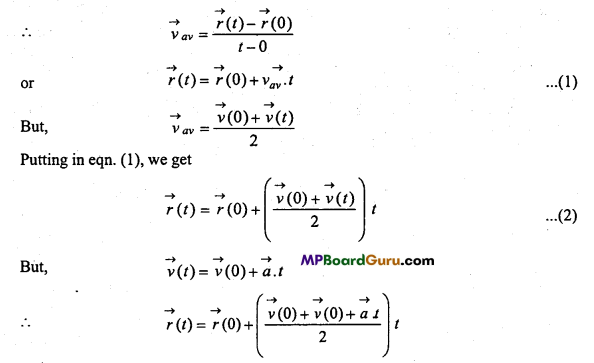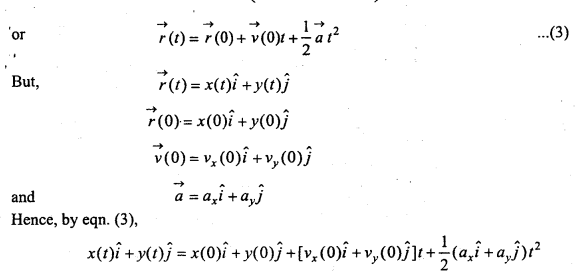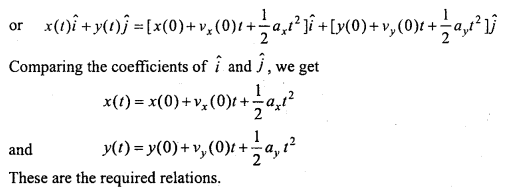Question 3.
What do you understand by projectile motion? Prove that the path of projectile is parabolic.
Projectile motion :
When a body is projected at an angle other than vertical, the body moves under gravity and falls at different points on the earth. The path traced out by the body is called trajectory and its motion is called projectile.
Example : Motion of cannon ball, the object released from an aeroplane flying horizontally. Trajectory is parabola when air of friction is negligible.

Let a body is projected with velocity u, at an angleθ with the horizontal. Thus, we have two components of u.
i.e., Horizontal component along X-axis = u cosθ
and Vertical component along T-axis = u sinθ
∵ The horizontal velocity is free from gravitational acceleration,
∴ ux = vx(0) = u cosθ and ax = 0
If x (t) is the position of the particle of any instant of time, then the displacement will be x(t) – λ(0),
But, λ(0) = 0
∴ x(t) – λ(0) = λ(t) = x
Hence, x = vx(0)t + $$\frac{1}{2}$$ axt2
or x = u cosθ t
or t = $$\frac{x}{u \cos \theta}$$ …(1)
Now, for the vertical component,
vy = u sinθ and ay = -g, (∵ the particle moves in upward direction) If y(t) is the position of the particle at any instant of time, then the displacement will be y(t) -y(0)
But, y(0) = 0
∴ y(t) – y(0)=y(t) = y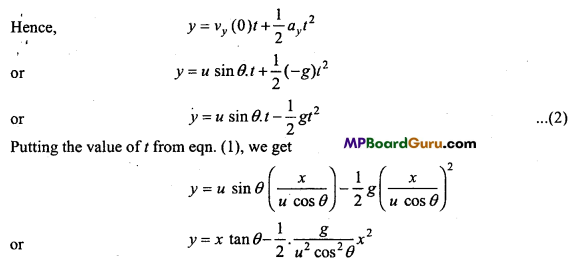or y = x tanθ –$$\frac{g}{2 u^{2} \cos ^{2} \theta} x^{2}$$ …(3)
Since, u, g and θ are constant, therefore the eqn. (3) is in the form of
y = ax – bx2
Where, a = tanθ and b = $$\frac{g}{2 u^{2} \cos ^{2} \theta}$$
Which is the equation of a parabola. Since, it is a quadratic equation in x. Proved.

Question 4.
Derive the expression for time of flight, horizontal range and maximum height attain for a projectile motion.
Expression for time of flight: Let a body is projected with initial velocity u at an angle θ with the horizontal.
∴ The components of u are:
(i) Horizontal component, ux = u cosθ and
(ii) Vertical component, uy = u Sinθ
The time taken by the projectile to travel from point O to A, is called its time of flight.
Now, initial velocity along Y-axis is
uyy(0)=u sinθ
Now,at highest point
νy(t) = 0 and ay = -g
If the time taken by the projectile from O to P is t, then
νy(t)= νy(0)+at
or
0=u sinθ-gt
or t = $$\frac{u \sin \theta}{g}$$
But Time of flight, T = 2t
∴ T = $$\frac{2 u \sin \theta}{g}$$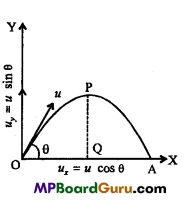Horizontal Range:
The horizontal distance travelled in the time of flight is called range.
Now, Range=OA=R
∴ R = Horizontal velocity × Time of flight
or R= u cosθ × $$\frac{2 u \sin \theta}{g}$$
or R= $$\frac{u^{2} \cdot 2 \sin \theta \cos \theta}{g}$$
∴ R = $$\frac{u^{2} \sin 2 \theta}{g}$$ (∵ 2sinθ cosθ = sin2θ)

For maximum range, sin2θ = 1
or sin2θ= sin9o°
or 2θ = 90°
∴ θ =45°
For maximum range the angle of projection should be 45°.
∴ Rmax = $$\frac{u^{2}}{g} .$$

Maximum height attain: Now, we have two resolved parts of initial velocity u, i,e.,
(i) Horizontal component ux = u cosθ and
(ii) Vertical component uy = u Sinθ.
Suppose, the maximum height of projectile is Hand it takes r sec. to reach the highest point.
Now, for vertical motion,
νy(0) = u sinθ and ay = -g
At highest point, νy(t) = 0
:. By the equation, νy(t)=νy(0)+ayt
or 0 = u sinθ – gt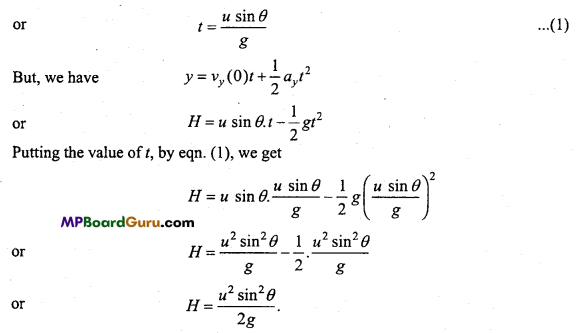Motion in a Plane Class 11 Important Numerical Questions

Question 1.
Find modulus and unit vector of the vector
$$\vec{a}=\hat{i}-2 \hat{j}+\hat{k}$$
Solution: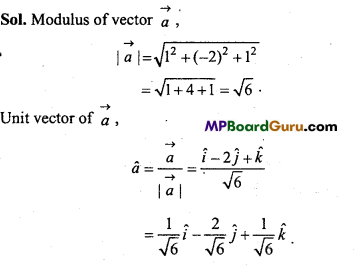Question 2.
If $$\vec{a}=\hat{i}+3 \hat{j}-4 \hat{k}$$ and $$\vec{b}=2 \hat{i}-3 \hat{j}+4 \hat{k}$$ then find out $$\vec{a}+\vec{b}$$,
$$\vec{a} \cdot \vec{b}$$ and $$\vec{a} \times \vec{b}$$
Solution: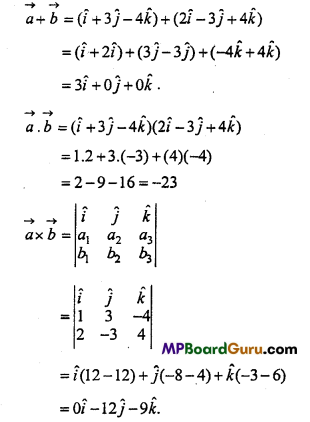Question 3.
If$$|\vec{a}+\vec{b}|=|\vec{a}-\vec{b}|$$, then prove that $$\text { a }$$ and $$\text { b }$$ are mutually perpendicular to each other.
Solution: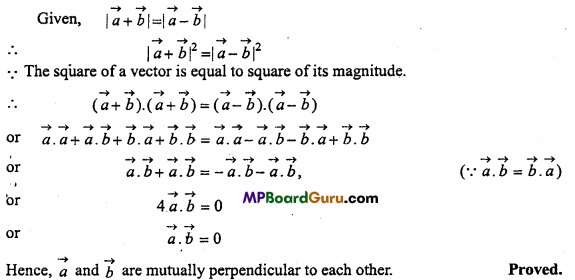Question 4.
When the resultant of two equal vectors will be A.
Solution:
R2 = A2 + B2 + 2ABcosθ
When $$\vec{B}=\vec{A}$$, then
R2 = A2 + A2 + 2A.Acosθ
= 2A2 +2A2cosθ
If costθ = – $$\frac{1}{2}$$ = cos 120°
Then R2 = A2
i.e., R = A
i.e., θ = 120°.

Question 5.
Prove that $$3 \hat{i}+2 \hat{j}-\hat{k}$$ and $$2 \hat{i}-2 \hat{j}+2 \hat{k}$$ are perpendicular to each other.
Solution: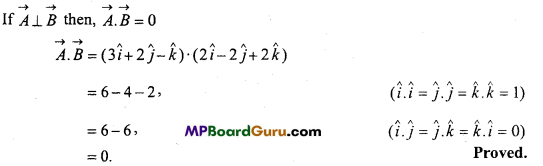Question 6.
If A = $$\vec{A}=2 \hat{i}+2 \hat{j}+p \hat{k}$$ and $$\vec{B}=2 \hat{i}-\hat{j}+\hat{k}$$are perpendicular, then find the value of p.
Solution:
Given : $$\vec{A}$$ and $$\vec{B}$$ are perpendicular
∴ $$\vec{A} \cdot \vec{B}$$ = 0
or $$(2 \hat{i}+2 \hat{j}+p \hat{k}) \cdot(2 \hat{i}-\hat{j}+\hat{k})$$ = 0
∴ 2.2 + 2.(-1) + p.(1) = 0
or 4 -2 + p = 0
or P = -2.

Question 7.
Rain is falling vertically with a speed of 30 ms *. A woman rides a bicycle
with a speed of 10 ms 1 in the north to south direction. What is the direction in which she should hold her umbrella?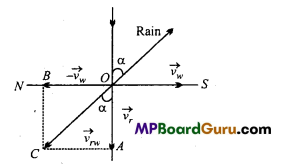Solution:
Let the rain be falling vertically downward with the speed of 30 ms-1 along OA. The woman is moving along OS with a speed of 10 ms-1.
To protect herself from rain, the woman should hold her umbrella in the direction of relative velocity of the rain with respect to the woman i.e., νrw. To determine the νrw the woman must be brought to rest by imposing an equal and opposite velocity of the observer both on woman as well as on the rain. Rain thus has two velocities :
(i) Its own velocity of 30 ms -1 along $$\overrightarrow{O A}$$
(ii) Imposed velocity of 10 ms-1 along $$\overrightarrow{O B}$$
The relative velocity is the resultant of these two velocities.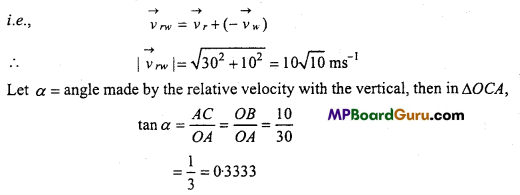tan α = tan18°26′
α = 18° 26′
So woman should hold umbrella at an angle of 18°26′ with the vertical towards south.Question 8.
A cyclist is riding with a speed of 27 kmh-1. As he approaches a circular turn on the road of radius 80 m, he applies brakes and reduces his speed at the constant rate of 0.5 ms-1 every second. What is the magnitude and direction of the net acceleration of the cyclist on the circular turn?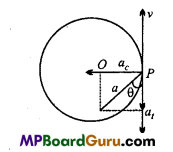Speed of cyclist ν = 27kmh-1
= 27 x $$\frac{5}{18}$$ ms-1
= $$\frac{15}{2}$$ ms-1
Radius of circular turn, r = 80 m
Here the cyclist will be acted upon by two accelerations ac and at Centripetal acceleration (ac) is given by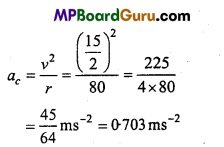Let P be the point at which the cyclist applies brakes, then the tangential acceleration at, (which will be negative) will act opposite to velocity of cyclist and is given by
at = $$\frac{\text { Change in velocity }}{\text { Time }}=\frac{0 \cdot 5}{1}$$ ms-2
= 0.5 ms-2
As both ac and at, act along the radius of circle and tangent to the circle respectively, so ac and a, are mutually perpendicular, thus the magnitude of the net acceleration ‘a’
(i.e., resultant acceleration) is given by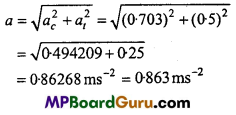Direction of a: Let θ be the angle made by the net acceleration with the velocity of cyclist, then
tanθ $$\frac{a_{c}}{a_{t}}$$ = $$\frac{0.703}{0.5}$$
= 1.406
or tanθ = tan54°33′
θ = 54° 33′ = 54-44°.

Question 9.
A ball is projected at an angle of 30° with a velocity of 20 ms-1. Find the time of flight.
Solution:
u = 20 ms-1, θ= 30°.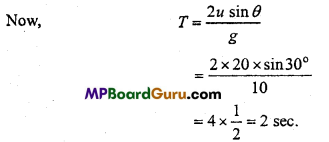Question 10.
A cricketor can throw a ball to a maximum horizontal distance of 100 m. How much high above the ground can the cricketor throw the same ball?
Solution:
Given:
R = 100 m
From the formula R = $$\frac{u^{2}}{g}$$, we get g
100 = $$\frac{u^{2}}{g}$$
and H = $$\frac{u^{2}}{2 g}=\frac{R}{2}=\frac{100}{2}$$ = 50m

Question 11.
A ball is projected at an angle of 60° with a velocity of 40 ms-1. What will be the maximum height and range?
Solution: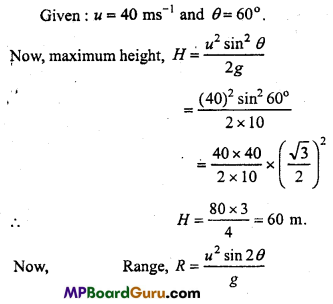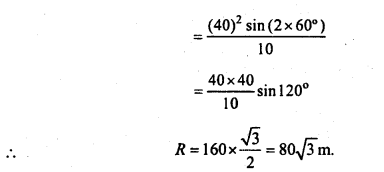Question 12.
A body is projected at an angle of 15° and its range is 50 m. If it is projected at an angle of 45°, then find the range.
Solution: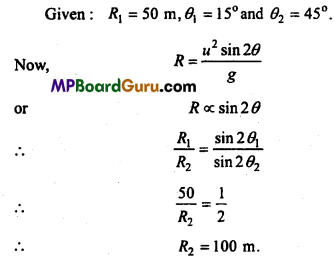Question 13.
A particle is executing 3 revolutions per second in a circular path of radius 15 cm. Find out angular velocity and linear velocity.
Solution:
Given r = 15 cm, frequency n = 3 revolutions per second
From ω = 2πn, we get
ω = 2 × π × 3 = 6π radian/sec.
Again, from ν = rω, we get
ν = 15 × 6π = 90πcm/sec.

Question 14.
In a projectile motion of the horizontal range and maximum height attain of an object is same, then find out the angle of projection of the object.
Solution:
Given, R = H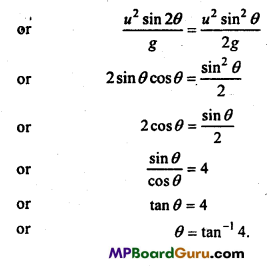Question 15.
The coordinate of a particle moving in a plane at any time f is given by JC =
ct2 and y = bt2 Determine the velocity of the particle.
Solution: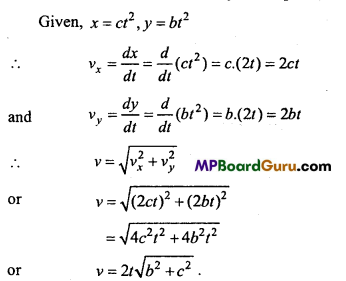Question 16.
For any projectile motion the horizontal range is four times of maximum height attain. Find out the angle of projection.
Solution:
Given R = 4 H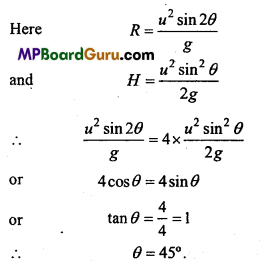Question 17.
A bullet is fired with a velocity of 1,000 m/s from a rifle at an angle of 30° w.r.t horizontal, find out:
(i) Time taken to reach highest point from the rifle.
(ii) Total time of flight
(iii) What will be the velocity of bullet when it strike ground surface?
(iv) At what height the buUet will raise ?
(v) At what distance from the rifle the bullet will strike the ground surface?
Solution: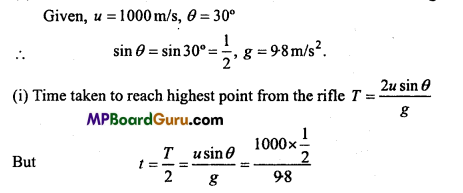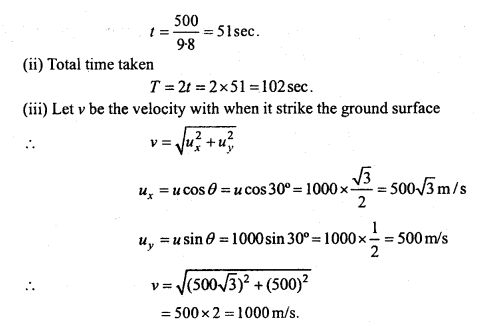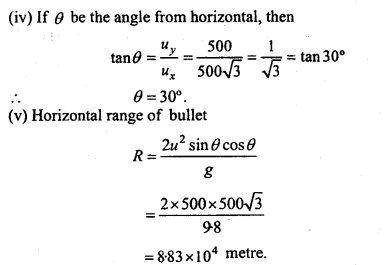Question 18.
A football player kicks the football with a velocity of 20 m/s making an angle of 30° from the horizontal. Find out
(i) Time of flight
(ii) Maximum height attain by the football
(iii) Velocity of the football and its direction after striking the ground.
(iv) Horizontal range, (given g = 10 m/s2).
Solution: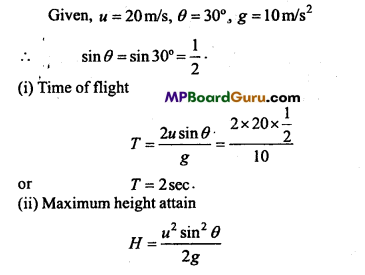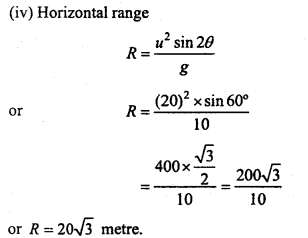Question 19.
An aeroplane is flying at a height of 1960 m with a velocity of 600 km/hr. When it is just above a point A on the earth, a packet is dropped, which falls at point B. Calculate AB.
Solution:
u = 600 km/hr =600 × $$\frac{5}{18}$$ m/s
= $$\frac{500}{3}$$ m/s
For motion of a packet in downward direction,
y = $$\frac{1}{2}$$ gt2
or 1960 =$$\frac{1}{2}$$ × 9.8 × t2
or t2 =400t = 20 sec.
∴ AB = ut = $$\frac{500}{3}$$ × 20
Horizontal distance covered by packet i.e.,AB
AB = ut = $$\frac{500}{3}$$ × 20
= 3333.3m
= 3.33km.Motion in a Plane Class 11 Important Questions Objective Type

1. Multiple- choice questions:

Question 1.
1. Torque is a :
(a) Vector quantity
(b) Scalar quantity
(c) Both
(d) None of these
(b) Scalar quantity

Question 2.
If $$\vec{A}=2 \hat{i}+4 \hat{j}$$and $$\vec{B}=2 \hat{i}-5 \hat{j}$$, then $$\vec{A}+\vec{B}$$ will be :
(a) $$5 \hat{i}-\hat{j}$$
(b) $$3 \hat{i}-\hat{j}$$
(c) $$4 \hat{i}-\hat{j}$$
(d) $$3 \hat{i}-3 \hat{j}$$
(c) $$4 \hat{i}-\hat{j}$$

Question 3.
Unit vector in direction of vector $$\vec{A}$$ will be :
(a) $$\hat{\mathrm{A}}=\frac{A}{\vec{A}}$$
(b) $$\hat{\mathrm{A}}=\frac{\vec{A}}{A}$$
(c) $$\text { (c) } \hat{\mathrm{A}}=A \cdot \vec{A}$$
(d) $$\text { (d) } \hat{\mathrm{A}}=A+\vec{A}$$
(b) $$\hat{\mathrm{A}}=\frac{\vec{A}}{A}$$

Question 4.
Angle between velocity and acceleration of maximum height of a projectile is :
(a) 30°
(b) 45°
(c) 60°
(d) 90°
(d) 90°

Question 5.
Path of a projectile is :
(a) Circular
(b) Straight line
(c) Parabolic
(d) Uncertain.
(c) Parabolic

Question 6.
For maximum range of a projectile, the angle must be :
(a) 30°
(b) 45°
(c) 60°
(d) 90°
(b) 45°

Question 7.
Time of flight of a projectile is tx and time taken to reach maximum height is t2, then the relation between them will be :
(a) t1 =2t2
(b) t2 =2t1
(c) t2 =3t1
(d) t 1=t2
(a) t1 =2t2

Question 8.
If the velocity of projectile is doubled, then its range will be :
(a) Same
(b) Become double
(c) Become half
(d) Become four times.
(b) Become doubleQuestion 9.
At maximum height of projectile, velocity is :
(a) Zero
(b) Minimum
(c) Equal to initial velocity
(d) None of these.
(b) Minimum

Question 10.
Rate of change of angular velocity is called :
(a) Angular displacement
(b) Angular speed
(c) Angular acceleration
(d) Angular momentum.
(c) Angular acceleration

2. Fill in the blanks:

1. Angular momentum is a ………………….quantity.
Vector

2. Magnitude of a vector $$2 \hat{i}-\hat{j}$$ is …………………….
√5

3. If an object is thrown horizontally from a height, then its path is ………………..
Parabolic

4. At maximum height of a projectile motion, velocity of an object is ………………..
Minimum

5. The maximum horizontal distance covered by a projectile in its time of flight is called ………………………
Horizontal range

6. ……………………….. is constant in uniform circular motion.
Speed

7. At maximum height the direction of acceleration in projectile motion is ………………….
Vertically downwards8. In uniform circular motion there is ……………………. changes in angular momentum of a particle.
Zero

9. Angle between velocity and acceleration in uniform circular motion is …………………..
90°

10. SI unit of angular momentum is …………………

3. Match the following:

I.

 Column ‘A’ Column ‘B’ 1. Formula for maximum height attain in projectile motion is (a) u cosθ 2. Formula for time of flight in projectile motion is (b) u sinθ 3. Formula for range in projectile motion is (c) $$\frac{u^{2} \sin 2 \theta}{2 g}$$ 4. Horizontal component if a particle moving with velocity u and making an angle θ with horizontal will be (d) $$\frac{2 u \sin \theta}{g}$$ 5. Vertical component if a particle moving with velocity u  and making an angle θ with horizontal will be (e) $$\frac{u^{2} \sin 2 \theta}{g}$$

1. (c) $$\frac{u^{2} \sin 2 \theta}{2 g}$$
2. (d) $$\frac{2 u \sin \theta}{g}$$
3. (e) $$\frac{u^{2} \sin 2 \theta}{g}$$
4. (a) u cosθ
5. (b) u sinθ

II.

 Column ‘A’ Column ‘B’ 1. Velocity of a particle performing uniform circular motion is (a) Tangential 2. Direction of acceleration of a particle performing uniform circular motion is (b) ν = rω 3. Relation between linear velocity (ν) and angular velocity (ω) is (c) Changes 4. Direction of velocity of a particle executing uniform circular motion is (d) Constant 5. Kinetic energy of a body executing uniform circular motion is (e) Toward centre.

1. (c) Changes
2. (e) Toward centre
3. (b) ν = rω
4. (a) Tangential
5. (d) Constant4. Write true or false:

1. If $$\vec{A} \cdot \vec{B}$$ = AB, then angle between $$\vec{A}$$ and $$\vec{B}$$ is zero.
True

2. If a unit vector is added or subtracted from a given vector, we always obtain given vector.
True

3. A scalar quantity can be added with vector quantity.
False

4. Resultant of three coplaner vector can be zero.
True

5. Path of motion is a straight line in two-dimensional motion moving with uniform velocity.
True

6. Acceleration is constant in projectile motion.
True

7. Horizontal velocity is constant in projectile motion.
True

8. If an object is dropped from running train, then its path will be parabolic.
True

9. Kinetic energy is zero at maximum height of a projectile motion.
False

10. In a given time interval, the ratio of change in velocity to the time-interval gives average acceleration.
True1. A projectile is thrown upwards. At the topmost point, the acceleration is in which direction.
Vertically downwards

2. When an object is projected from earth surface, then which quantity remains constant?